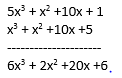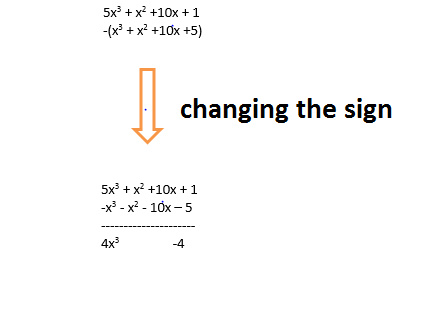Welcome to Class 9 Maths chapter 2 Polynomials notes.The topics in this page are adding polynomials and subtracting polynomials along with examples, and questions and answers .This is according to CBSE and the NCERT textbook. If you like the study material, feel free to share the link as much as possible.

Table of Content

1. Arrange both the polynomial is same order of exponent . It would be good to have terms arrange from highest exponent to lowest exponent i.e
For example
$S(x) = x^2 + 5x^3 + 1 +10x$
$P(x) = x^3 + x^2 + 5 +10x$

Arranging them as per suggestion above

$S(x) = 5x^3 +x^2+ 10x +1$
$P(x) = x^3 + x^2 + 10x +5$

2. Add the like term . By like term ,we mean same exponent terms. There are two method to add like terms. We either can group them horizontally and add it. We can add vertically
Horizontally
$S(x) + P(x)$
$=x^2 + 5x^3 + 1 +10x + (x^3 + x^2 + 5 +10x)$
Arranging both the polynomial in same order
$=5x^3 +x^2+ 10x +1 + (x^3 + x^2 + 10x +5 )$
Opening the parenthesis and grouping the like terms. In addition,no sign are changed when parenthesis are opened
$= 5x^3 + x^3 + x^2+x^2 + 10x +10x + 1+5$
$=6x^3+2x^2+20x+6$

Vertically
$S(x) = x^2 + 5x^3 + 1 +10x$
$P(x) = x^3 + x^2 + 5 +10x$

Arranging both the polynomial in same order

$S(x) = 5x^3 +x^2+ 10x +1$
$P(x) = x^3 + x^2 + 10x +5$Similarly we can add three or more polynomials

Example-1
$(2x^3 - x+1+x^2) + (x^3 + 6x - 7) + (-3x^2 - 11 + 2x)$
Arranging them in same order
$=(2x^3 + x^2 - x+1) + (x^3 + 6x - 7) + (-3x^2 + 2x - 11)$

Opening the parenthesis and grouping the like terms
$=2x^3+x^3 +x^2-3x^2 -x +6x+2x +1-7-11$
$=3x^3-2x^2+7x-16$

## Subtracting polynomials

We many times need to subtract the two polynomial .It is very similar to adding polynomials only.Subtracting polynomials just means Subtracting the like terms.We need to follow below steps for Subtraction of polynomial
1. Arrange both the polynomial is same order of exponent . It would be good to have terms arrange from highest exponent to lowest exponent i.e
For example
$S(x) = x^2 + 5x^3 + 1 +10x$
$P(x) = x^3 + x^2 + 5 +10x$

Arranging them as per suggestion above

$S(x) = 5x^3 +x^2+ 10x +1$
$P(x) = x^3 + x^2 + 10x +5$

2. Subtract the like term . By like term ,we mean same exponent terms. There are two method to subtract like terms. We either can group them horizontally and subtract it. We can add vertically
Horizontally
$S(x) - P(x)$
$=x^2 + 5x^3 + 1 +10x - (x^3 + x^2 + 5 +10x)$
Arranging both the polynomial in same order
$=5x^3 +x^2+ 10x +1 -(x^3 + x^2 + 10x +5 )$
Opening the parenthesis and grouping the like terms. In Subtract, sign are reversed when parenthesis are opened i.e + becomes - and - becomes +
$= 5x^3 - x^3 + x^2 - x^2 + 10x -10x + 1-5$
Subtract the like term
$=4x^3 - 4$

Vertically
$S(x) = x^2 + 5x^3 + 1 +10x$
$P(x) = x^3 + x^2 + 5 +10x$

Arranging both the polynomial in same order

$S(x) = 5x^3 +x^2+ 10x +1$
$P(x) = x^3 + x^2 + 10x +5$Similarly we can add three or more polynomials

Example-1
$(2x^3 - x+1+x^2) - (x^3 + 6x - 7) - (-3x^2 - 11 + 2x)$
Arranging them in same order
$=(2x^3 + x^2 - x+1) - (x^3 + 6x - 7) - (-3x^2 + 2x - 11)$

Opening the parenthesis and grouping the like terms
$=2x^3- x^3 +x^2+3x^2 -x -6x-2x +1+7+11$
$=x^3+4x^2-9x+18$

## Practice Problems

(i) $x^2 + 5x + 1$ and $x^2 - 4x - 1$
(ii) $2x^3 + 5x^2 + 11x + 99$ and $-x^3 + 4x - 1+ 5x^2$
(iii) $x^2 - 5x^3 + 110x+ 1$ and $x^3 - 4x^4 - 1-x$
(iv) $-x^5 + 5x^2 + 9x^3 + 11$ and $4x^5 - 4x^2 - x^2+8x + 9$
(v) $x^2 + x^4 + 1$ , $x^2 - 4x - 1$ and $x^3 - 4x^4 - 1-x$
(vi) $x^3 + 5x + 1$ ,$-x^3 - 4x - 1$ and $-2x^3 + 4x - 1+ 5x^2$
(vii) $x^3 + 55x + 10x-5$ , $x^4 - 4x - 1$ ,$-x^4 - 4x - 1$ and $-2x^4 + 4x - 1+ 5x^2$
Subtract the polynomials
(i) $3x^4 -4x^3 + 2x^2 - 6x + 6$ from $-4x^4 + 8x^3 - 4x^2 –x + 11$
(ii) $-x^5 + 5x^2 + 9x^3 + 11$ from $4x^5 - 4x^2 - x^2+8x + 11$
(iii) $x^3 + x^4 - 1$ from $-x^3 - 4x^4 - 1-x$
(iv) $2x^3 - 5x^2 + 11x + 99$ and $x^3 + 4x - 1- 5x^2$